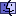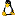Making a Single Color Transparent Using MaskDataUPDATE 7/29/02: The MetaCard 2.4.3 engine (used by MetaCard 2.4.3 and Revolution 1.5A7 or later) fixed a bug that relating to the order of the color bytes in `imageData`.

 The Tip on this page is based on the MetaCard 2.4.3 engine. If you have a version of MetaCard/Revolution that uses an earlier engine than 2.4.3, you can view the original Tip for assistance.

This assumes you have a good understanding of how to manipulate `imageData` and `maskData`. If you don’t, take a look at tip imag003 - Understanding ImageData, MaskData and AlphaData before continuing.

The following is an example of creating a single-color transparency of an image much like what is used in GIF images. I have chosen to use pure red (RGB: 255,0,0) as the mask color.

```on mouseUp
put the imageData of image 1 into iData
put the width of image 1 into tW
put the height of image 1 into tH
put empty into mData

repeat with i = 1 to tH  -- iterate each row
repeat with j = 1 to tW   -- iterate each column
-- Get a pointer to the specific end byte of a pixel
-- Remember there are 4 bytes per pixel
put ((i-1)*tW*4)+(j*4) into tByte
-- Go backwards from the end byte to get your R, G and B
put charToNum(char (tByte-2) of iData) into tR
put charToNum(char (tByte-1) of iData) into tG
put charToNum(char (tByte) of iData) into tB
if (tR = 255) and (tG = 0) and (tB = 0) then
-- pixel is red, mask it
put binaryEncode("C",0) after mData
else
put binaryEncode("C",255) after mData
end if
end repeat
end repeat

set the maskData of image 1 to mData
set the imageData of image 1 to iData
end mouseUp```
Hope you find this as useful as I did. :-)

Posted 7/2/2002 by Ken Ray